# Chapter 11 Trigonometric Identities and Equations 11 1

• Slides: 11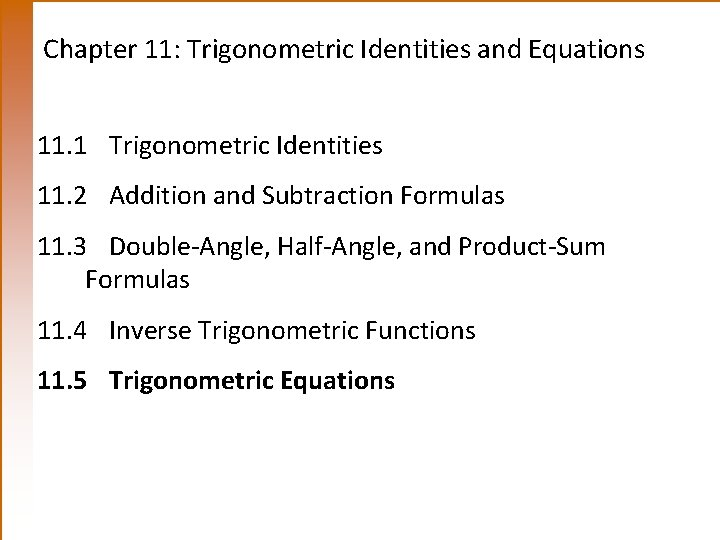Chapter 11: Trigonometric Identities and Equations 11. 1 Trigonometric Identities 11. 2 Addition and Subtraction Formulas 11. 3 Double-Angle, Half-Angle, and Product-Sum Formulas 11. 4 Inverse Trigonometric Functions 11. 5 Trigonometric Equations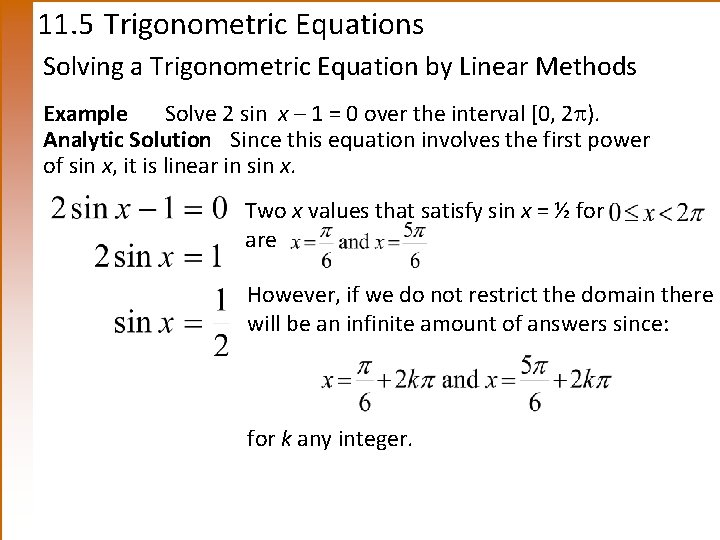11. 5 Trigonometric Equations Solving a Trigonometric Equation by Linear Methods Example Solve 2 sin x – 1 = 0 over the interval [0, 2 ). Analytic Solution Since this equation involves the first power of sin x, it is linear in sin x. Two x values that satisfy sin x = ½ for are However, if we do not restrict the domain there will be an infinite amount of answers since: for k any integer.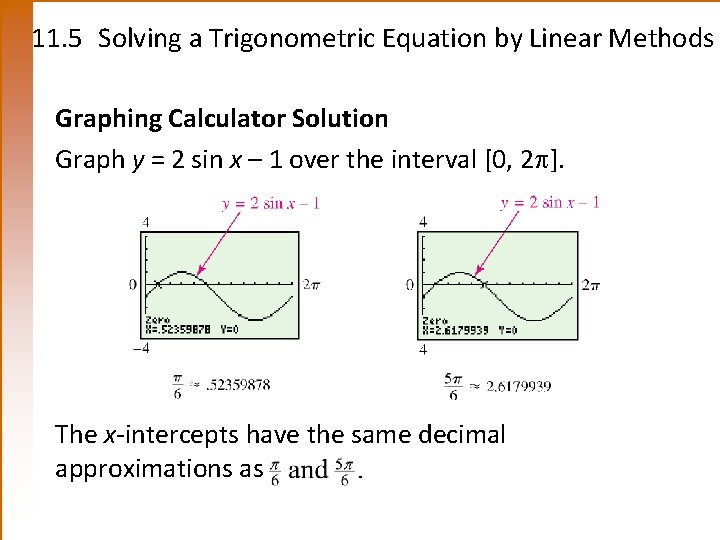11. 5 Solving a Trigonometric Equation by Linear Methods Graphing Calculator Solution Graph y = 2 sin x – 1 over the interval [0, 2 ]. The x-intercepts have the same decimal approximations as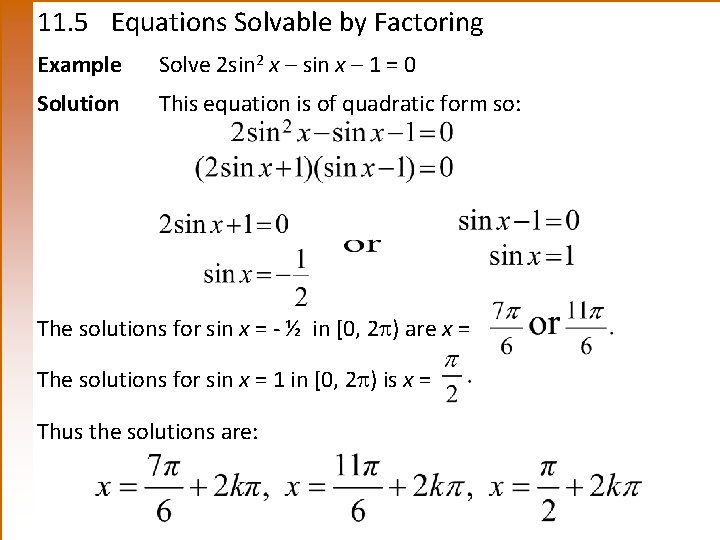11. 5 Equations Solvable by Factoring Example Solve 2 sin 2 x – sin x – 1 = 0 Solution This equation is of quadratic form so: The solutions for sin x = - ½ in [0, 2 ) are x = The solutions for sin x = 1 in [0, 2 ) is x = Thus the solutions are: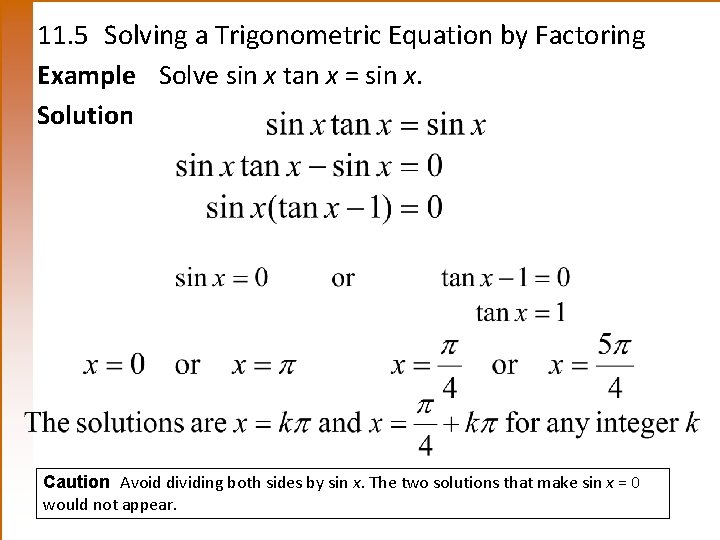11. 5 Solving a Trigonometric Equation by Factoring Example Solve sin x tan x = sin x. Solution Caution Avoid dividing both sides by sin x. The two solutions that make sin x = 0 would not appear.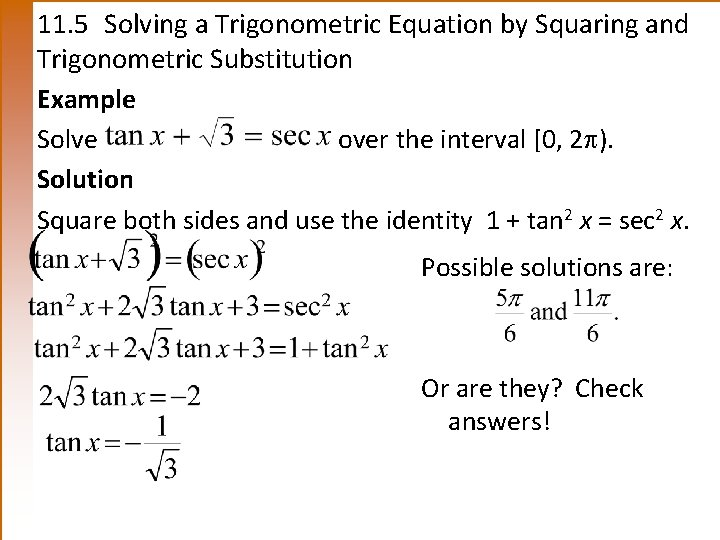11. 5 Solving a Trigonometric Equation by Squaring and Trigonometric Substitution Example Solve over the interval [0, 2 ). Solution Square both sides and use the identity 1 + tan 2 x = sec 2 x. Possible solutions are: Or are they? Check answers!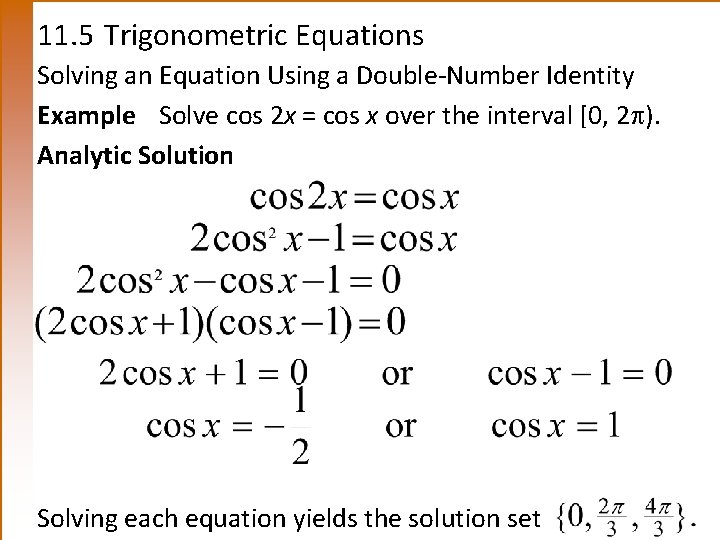11. 5 Trigonometric Equations Solving an Equation Using a Double-Number Identity Example Solve cos 2 x = cos x over the interval [0, 2 ). Analytic Solution Solving each equation yields the solution set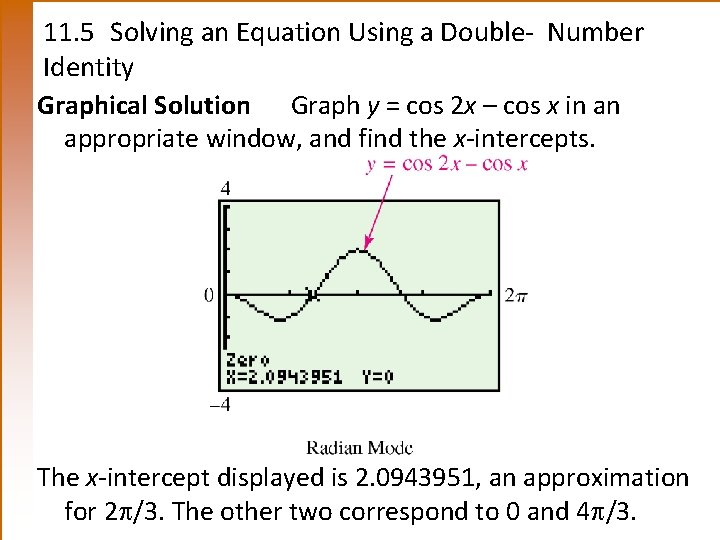11. 5 Solving an Equation Using a Double- Number Identity Graphical Solution Graph y = cos 2 x – cos x in an appropriate window, and find the x-intercepts. The x-intercept displayed is 2. 0943951, an approximation for 2 /3. The other two correspond to 0 and 4 /3.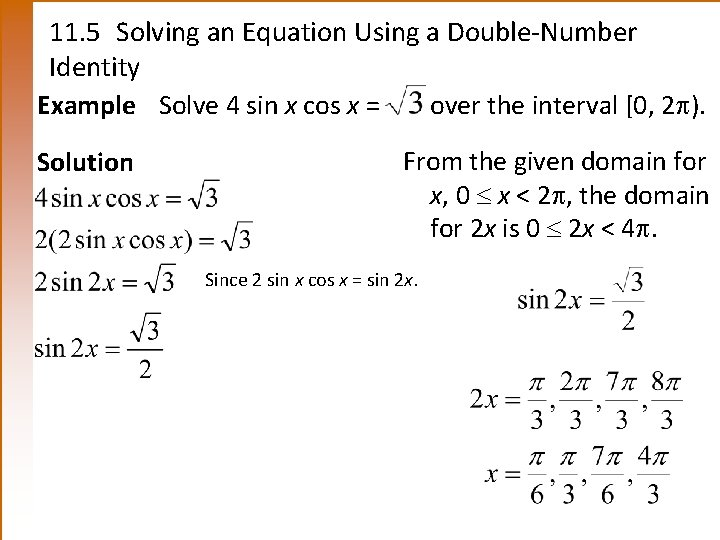11. 5 Solving an Equation Using a Double-Number Identity Example Solve 4 sin x cos x = over the interval [0, 2 ). Solution From the given domain for x, 0 x < 2 , the domain for 2 x is 0 2 x < 4. Since 2 sin x cos x = sin 2 x.11. 5 Solving an Equation that Involves Squaring Both Sides Example Solve tan 3 x + sec 3 x = 2 Solution Since the tangent and secant functions are related by the identity 1 + tan 2 = sec 2 , we begin by expressing everything in terms of secant. Square both sides. Replace tan 2 3 x with sec 2 3 x – 1.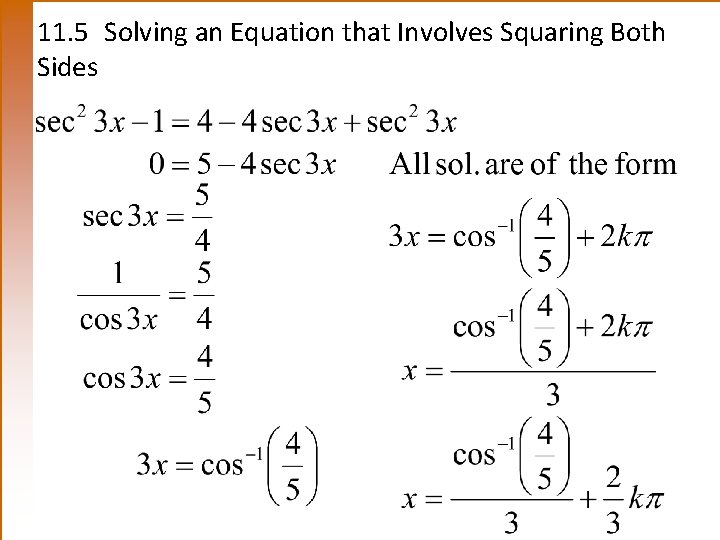11. 5 Solving an Equation that Involves Squaring Both Sides